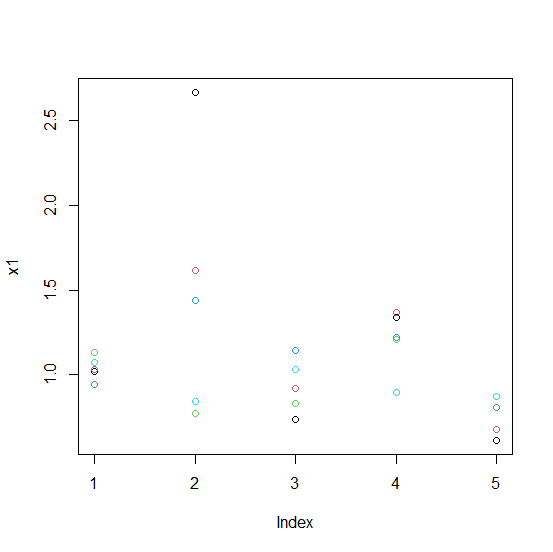# How to create a scatterplot using five vectors in a single plot window without separating the plots in R?

To create more than one scatterplot in a single plot window we should create the scatterplot for first vector and then add the point of the remaining vectors by using points function and they can be displayed with different colors so that it becomes easy to differentiate among the points of the vectors.

## Example

Consider the below vectors −

Live Demo

x1<-rnorm(5,1,0.5)
x2<-rnorm(5,1,0.4)
x3<-rnorm(5,1,0.3)
x4<-rnorm(5,1,0.2)
x5<-rnorm(5,1,0.1)
plot(x1)

## Output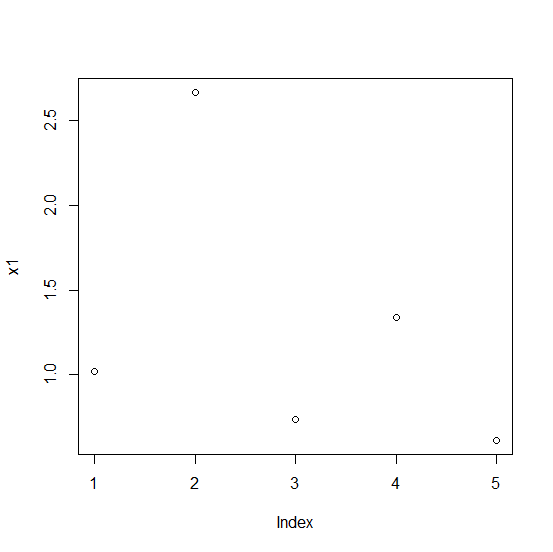## Example

points(x2,col=2)

## Output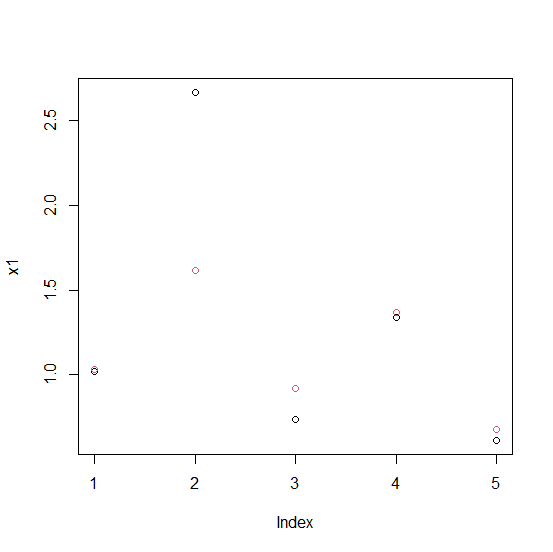## Example

points(x3,col=3)

## Output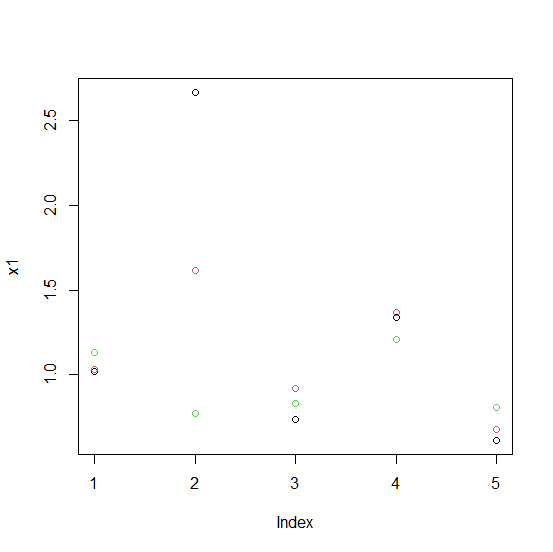## Example

points(x4,col=4)


## Output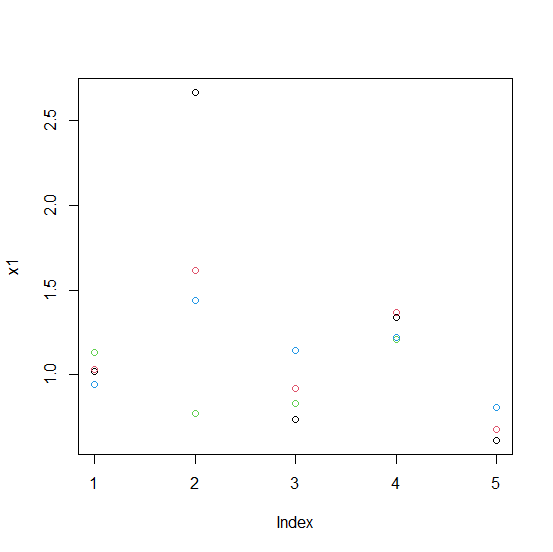## Example

points(x5,col=5)

## Output